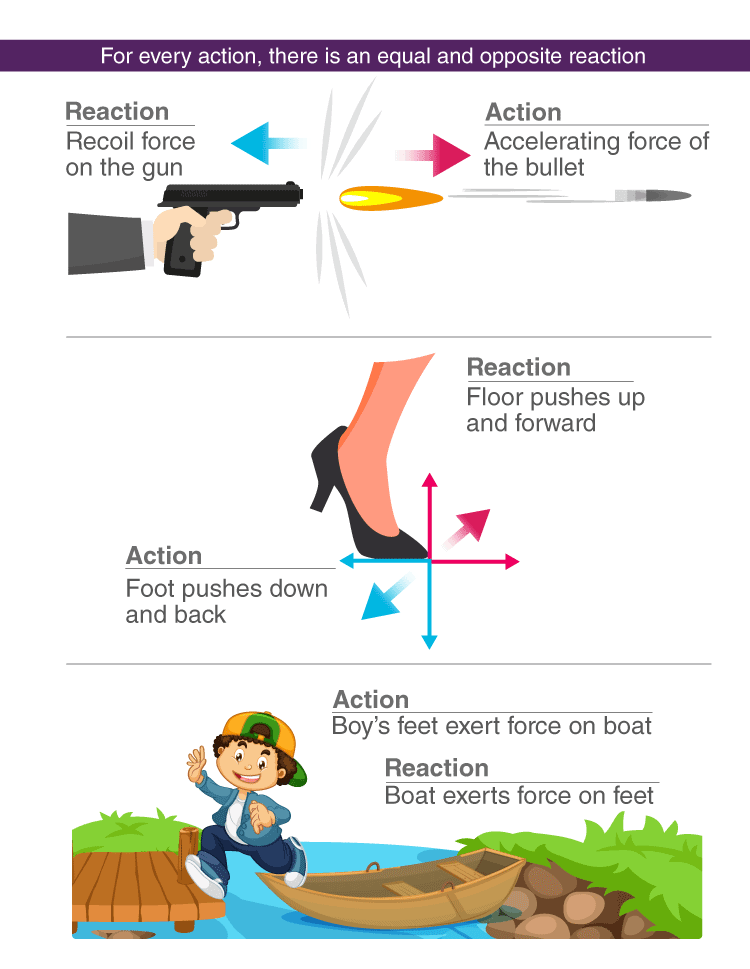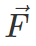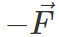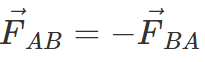Courses

# Third Law of Motion Class 11 Notes | EduRev

## NEET : Third Law of Motion Class 11 Notes | EduRev

The document Third Law of Motion Class 11 Notes | EduRev is a part of the NEET Course Physics Class 11.
All you need of NEET at this link: NEETIntroduction

You probably know that when you throw a ball against a wall, the ball exerts a force on the wall. Likewise, the wall puts force on the ball as a result of which the ball bounces off the wall. Similarly, earth pulls you down with gravitational force. What you may not realize is you are also exerting an equal amount of force on the earth. This remarkable fact is a consequence of Newton’s third law.

Newton’s 3rd Law: If an object A exerts a force on object B, then object B must exert a force of equal magnitude and opposite direction back on object A.
This law signifies a particular symmetry in nature: forces always occur in pairs, and one body cannot exert a force on another without experiencing a force itself. In the next few sections, let us learn Newton’s third law in detail.

Newton’s Third Law Of Motion

Force is a push or pull acting on an object resulting in its interaction with another object. Force is a result of an interaction. Force can be classified into two categories: contact force such as frictional force and non-contact force such as gravitational force. According to Newton, when two bodies interact, they exert force on each other, and these forces are known as action and reaction pairs, which is explained in Newton’s third law of motion. Newton’s third law of motion states that

“When one body exerts a force on the other body, the first body experiences a force which is equal in magnitude in the opposite direction of the force which is exerted”.

The above statement means that in every interaction, there is a pair of forces acting on the interacting objects. The magnitude of the forces are equal and the direction of the force on the first object is opposite to the direction of the force on the second object.

Solved Example

Aswini and Deepa are standing on identical skateboards. Aswini and Deepa push off of each other and travel in opposite directions. If Aswini (M) and Deepa (m) have identical masses, who travels farther?
Answer: Neither. Both will travel the same distance because the force applied to each will be the same.
Ma = ma
a = a
Acceleration controls how far each of them will travel. Since both have the same acceleration, they travel equal distance.
The mathematical representation of Newton’s third law of motion is let A be the body exerting forceon the body B, then body B too exerts a forceon body A, which is given as:Newton’s third law of motion is associated with conservation of momentum. According to the law for every action, there must be an equal and opposite reaction.

Examples of Interaction Force Pairs

A variety of action-reaction pairs are evident in nature. We have listed a few below, and they are as follows:

• Propulsion of fish through water is an example of an action-reaction pair. A fish makes use of its fins to push water backwards. This push serves to accelerate the fish forwards. The size of the force on the water equals the size of the force on the fish; the direction of the force on the water (backwards) is opposite the direction of the force on the fish (forwards).
• The flight of the bird is an example of an action-reaction pair. The wings of the bird push the air downwards. The air pushes the air upwards.
• A swimmer pushes against the water, while the water pushes back on the swimmer.
• Lift is created by helicopters by pushing the air down, thereby creating an upward reaction force.
• Rock climbers pull their vertical rope downwards to push themselves upwards.
Offer running on EduRev: Apply code STAYHOME200 to get INR 200 off on our premium plan EduRev Infinity!

## Physics Class 11

75 videos|312 docs|152 tests

,

,

,

,

,

,

,

,

,

,

,

,

,

,

,

,

,

,

,

,

,

;